# What is Wave Propagation? Definition, Equation, and Its Types

Wave is a disturbance that transfers energy through medium or space with negligible or no amount of mass transfer. There are various types of waves which render many different types of services. Electromagnetic waves are widely used in engineering applications. We use waveforms in various types of applications such as wireless communication, Radar, Space Exploration, Marine, Radio navigation, Remote sensing etc…Among these applications, some uses guided medium for sending waves whereas some make use of the unguided medium. In this article, we would know how the properties of medium affect the propagation of waves and various ways in which a wave propagates.

## What is Wave Propagation? – Definition

Electromagnetic Waves are generated by the radiated power from the current carrying conductor. In conductors, a part of the generated power escapes and propagates into free space in the form of Electromagnetic wave, which has a time-varying electrical field, magnetic field, and direction of propagation orthogonal to each other.

Radiated from an isotropic transmitter, these wave travels through different paths to reach the receiver. The path taken by the wave to travel from the transmitter and reach the receiver is known as Wave Propagation.

### Electromagnetic (EM) or Radio Wave Propagation

When the isotropic radiator is used for transmission of EM waves we get spherical wavefronts as shown in the figure because it radiates EM waves uniformly and equally in all directions. Here the center of the sphere is the radiator while the radius of the sphere is R. Clearly, all the points at the distance R, lying on the surface of the sphere have equal power densities.

The E waves travel in the free space with the velocity of light .i.e. c =  But EM waves travel through another medium the speed reduces. The speed of EM waves in any medium other than free space is given by,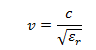where c is the velocity of light and is relative permittivity of the medium.

EM waves transmit energy by absorption and re-emission of wave energy by the atoms in the medium. The atoms absorb the wave energy, undergo vibrations and pass the energy by re-emission of EM of the same frequency. The optical density of the medium affects the propagation of EM waves.

### Wave Propagation Equation

Waves take many routes on their way to reach the receiver. Many parameters decide the path taken by the wave such as heights of the transmitting and receiving antennas, the angle of launching at transmitting end, the frequency of operation polarization etc…

Many of the properties of the waves get modified during propagation such as reflection, refraction, diffraction etc…due to the variation of parameters of propagating media like conductivity, permittivity, permeability, and characteristics of obstructing objects.

Generally, when power is radiated in the free space, the wave energy may be radiated or absorbed by the objects in the medium. So while transmitting a wave through a medium it is essential to calculate the loss that can be caused to the wave. This loss is called Radio transmission loss, which is based on inverse square law of optics and is calculated as the ratio of the radiated power to the received power.

As we know that when an isotropic transmitter is used, power is distributed equally, average power can be expressed in terms of radiated power as,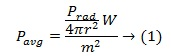The directivity of a test antenna is given by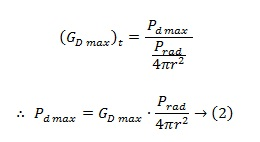Assume that the receiving antenna receives all the generated power from the radio waves without any loss. Let be the maximum power received by the receiver antenna under a matched load condition. When  is the effective aperture of the receiving antenna, we can write as,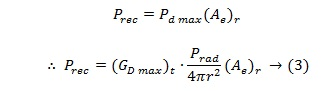In general, the directivity and effective aperture area for any antenna is related as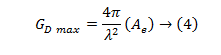Let be the directivity of the receiving antenna. Then,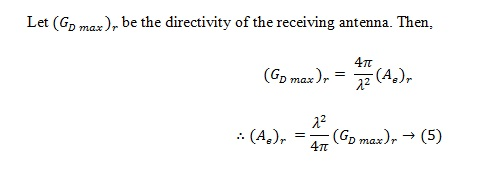Substituting the value in (3) we get,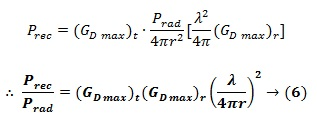This equation is known as the Fundamental Equation for free space Propagation, also known as Friss free space equation. The factor (λ/4πr)is called free space path loss which indicates the loss of the signal. Path loss can be expressed as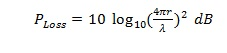We can express the equation (6) in dB as,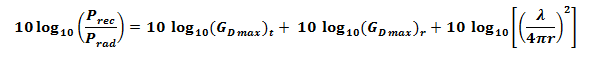Received power can be expressed as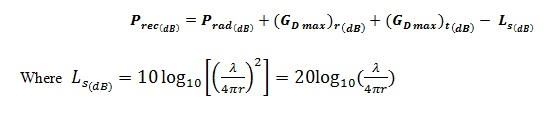Which, on simplification is given as,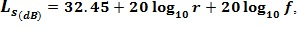Here distance r is expressed in kilometer while frequency f is expressed in MHz. This indicates loss due to wave spreading taking place when it propagates out of the source.

### Types of Wave Propagation

The electromagnetic waves or radio waves propagation, passing through the environment of the earth depend not only on the properties of themselves but also on the properties of the environment. There are different paths of propagation by which the transmitted waves can reach the receiver. All these modes depend on the frequency of operation, the distance between transmitter and receiver etc…

• The waves that propagate near the earth’s surface are called GROUND WAVES. This type of propagation is possible when the transmitting and receiving antenna both are closed to the earth’s surface.
• The ground waves which travel without any reflection are called Direct waves or Space waves.
• The ground waves which propagate to the receiving antenna through the reflection from the earth’s surface are called Ground Reflected waves or Surface waves.
• The waves which reach the receiving antenna due to scattering and reflection by the ionization in the upper atmosphere are called Skywaves.
• The waves which are reflected or scattered in the troposphere before reaching antenna are called troposphere waves.

#### Ground Wave or Surface Wave Propagation

A ground wave travels along the surface of the earth. These waves are vertically polarized. So, vertical antennas are useful for these waves. If a horizontally polarized wave is propagated as a ground wave, due to the conductivity of the earth, the electric field of the wave gets short-circuited.

As the ground wave travels away from the transmitting antenna it gets attenuated. To minimize this loss the transmission path must be over the ground with high conductivity. With respect to this condition, sea water should be the best conductor but it was observed that large storage of water in ponds, sandy or rocky soil shows maximum losses.

Hence, high power low-frequency transmitters, using ground wave propagations, are preferably located on ocean fronts. As ground losses increase rapidly with frequency, this propagation is used practically for signals up to frequency 2 MHz only.

For medium wave broadcast although ground waves are preferred some energy is transmitted to the ionosphere. But during day time the energy is completely absorbed by the ionosphere and during night time ionosphere reflects energy back to earth. So all the broadcast signal received during day time are due to ground wave only.

The maximum range of ground wave propagation not only depends on the frequency but also on the power of the transmitter. As ground waves pass over the surface of the earth they are also called the Surface wave.

#### SkyWave Propagation

Every long radio communication of medium and high frequencies are conducted using skywave propagation. In this mode reflection of EM waves from the ionized region in the upper part of the atmosphere of the earth is used for transmission of waves to longer distances.

This part of the atmosphere is called ionosphere which is at about 70-400 km height. Ionosphere reflects back the EM waves if the frequency is between 2 to 30 MHz’s. Hence, this mode of propagation is also called as Short wave propagation.

Using sky wave propagation point to point communication over long distances is possible. With the multiple reflections of sky waves, global communication over extremely long distances is possible.

But a drawback is that the signal received at the receiver has faded due to a large number of waves following a large number of different paths to reach the receiving point.

#### Space Wave Propagation

When we are dealing with EM waves of frequency between 30 MHz to 300 MHz, then space wave propagation is useful. Here properties of Troposphere are used for transmission.

When operating in space wave propagation mode, the wave reaches the receiving antenna directly from the transmitter or after reflection from troposphere which is present at about 16km above the earth surface. Hence space wave mode consists of two components .i.e. direct wave and indirect wave.

Though these components are transmitted at the same time with the same phase they may reach within the phase or out of phase with each other at the receiver end depending on the different path lengths. Thus, at the receiver side signal strength is the vector sum of strengths of direct and indirect waves.

The space wave propagation mode is used for propagation of very high frequencies.

#### Which of The Propagation Is Used For Short Wave Broadcasting

Short wave broadcasting usually takes place in the frequency range of 1.7 – 30 MHz. As we have seen above the frequencies in this range are propagated through Skywave propagation mode.

Depending on the frequency or wavelength the electromagnetic waves produce different affected in various materials and devices. Hence, the different parts of the electromagnetic spectrum are utilized for various applications. Which of the wave propagation intrigues you? Application of which of the propagation mode you find challenging.